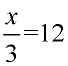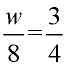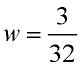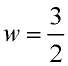Practice with Basic Linear Equations Terms of Use    Contact Person: Donna RobertsDirections: You may need paper and pencil. Choose the correct answer.

1.
Solve for x:   x + 8 = 19Choose:
 x = 27 x = -27 x = 11 x = -11

2.
Solve for m:   m - 6 = 24Choose:
 m = 30 m = -30 m = 18 m = -18

3.
Solve for x:   x - 12 = -16Choose:
 x = 28 x = -28 x = 4 x = -4

4.
Solve for q:   5q = 125Choose:
 q = 120 q = 130 q = 25 q = 625

5.
Solve for x:Choose:
 x = 4 x = 9 x = 15 x = 36

6.
Solve for a:   9 + a = 30Choose:
 a = 39 a = -39 a = 21 a = -21

7.
Ten times a number, n, is 530. Which equation can be used to find n?Choose:
 10n = 530 10 = n + 530 10 + n = 530 n = 10/530

8.
A number, n, decreased by 14 equals 60. Which equation can be used to find n?Choose:
 n = 60 - 14 n + 14 = 60 14n = 60 n - 14 = 60

9.
Solve for x:   x + 4.5 = -8.7Choose:
 x = 13.2 x = -13.2 x = 4.2 x = -4.2

10.
Solve for w:Choose:
 w = 2 w = 6